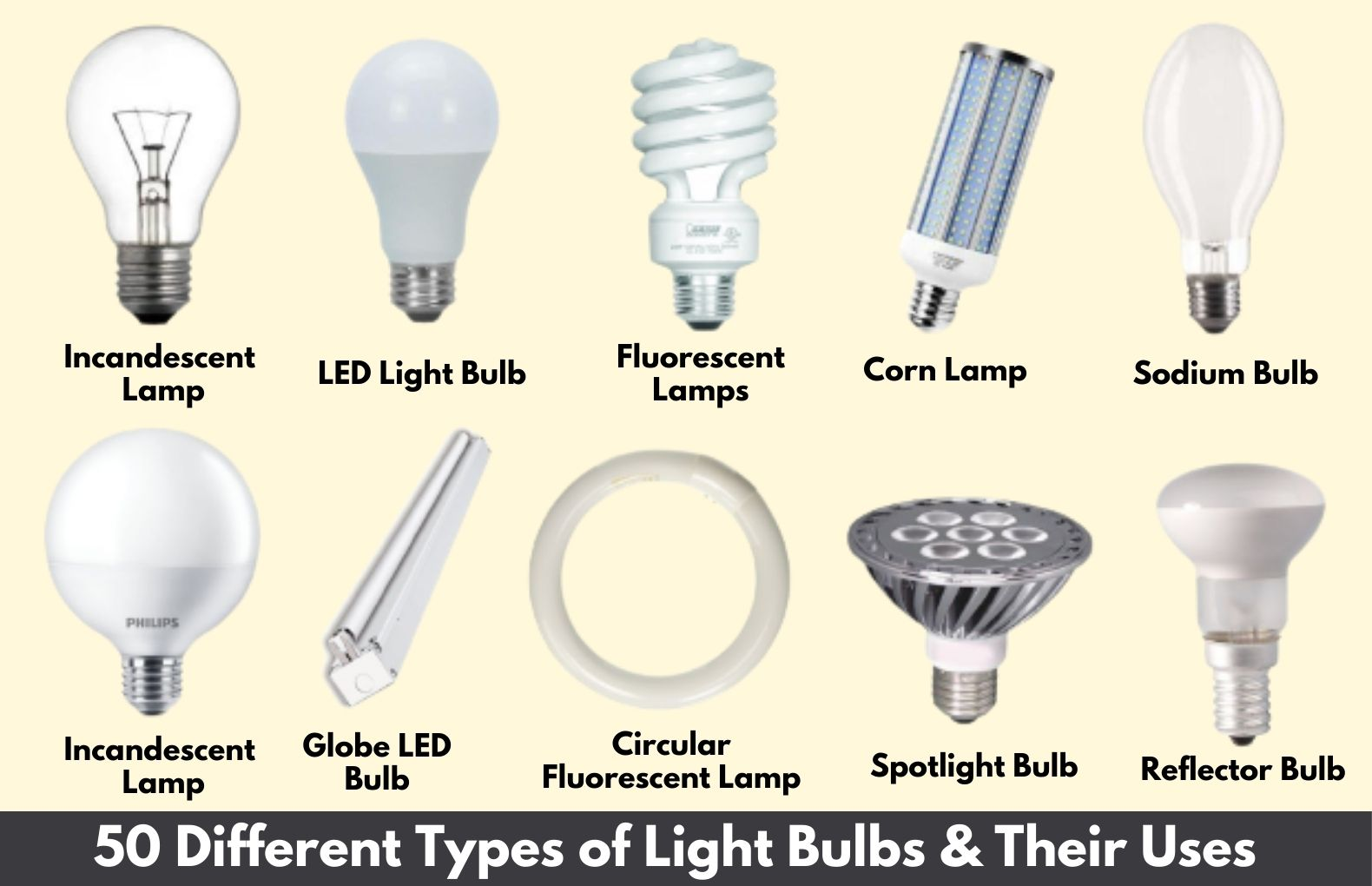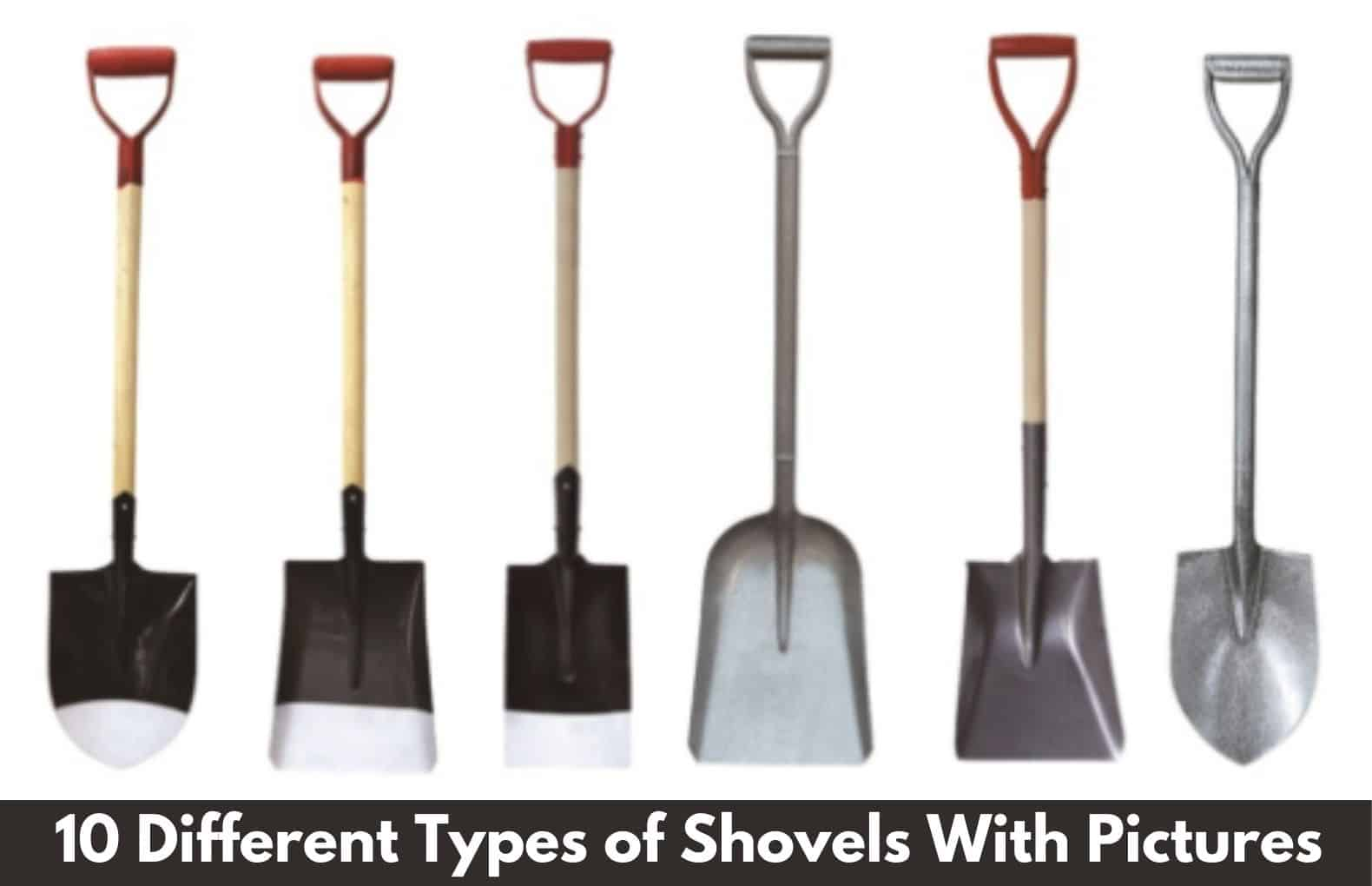# Structural Analysis MCQ

Civil engineering MCQ and structural analysis MCQ for competitive exam.

A. 3

B. 5

C. 4

D. 6

A. 3

A. m ‐ r + 2j

B. m + r ‐ 2j

C. m ‐ r + 2j

D. m + r + 2j

B. m + r ‐ 2j

A. 5

B. 3

C. 2

D. 1

B. 3

A. 6

B. 5

C. 1

D. 4

A. 6

A. 2j ‐ r

B. j ‐ 2r

C. 3j ‐ r

D. 2j + r

A. 2j ‐ r

## Q. 6 Independent displacement components at each joint of a rigid‐jointed plane frame are

A. three linear movements

B. two linear movements and one rotation

C. one linear movement and two rotationsc

D. three rotations

B. two linear movements and one rotation

## Q. 7If in a pin‐jointed plane frame (m + r) > 2j, then the frame is

A. stable and statically determinate

B. stable and statically indeterminate

C. unstable

D. none of the above

where m is number of members, r is reaction components and j is number of joints

B. stable and statically indeterminate

## Q. 8 A pin‐jointed plane frame is unstable if

A. (m + r)<2j

B. m + r = 2j

C. (m + r)>2j

D. none of the above

where m is number of members, r is reaction components and j is number of joints

A. (m + r)<2j

## Q. 9 A rigid‐jointed plane frame is stable and statically determinate if

A. (m + r) = 3j

B. (3m + r) = 3j

C. (m + r) = 2j

D. (m + 3r) = 3j

where m is number of members, r is reaction components and j is number of joints

C. (m + r) = 2j

## Q. 10 The number of independent equations to be satisfied for static equilibrium in a space structure is

A. 5

B. 3

C. 6

D. 2

C. 6

### Popular Post on Website#### Types of Architects: Exploring the Different Roles and Specializations in Architecture#### Types of Crown Molding: Explore 11 Different Styles and Materials for Elegant Home Décor#### Types of Light Bulbs: A Comprehensive Guide to 50 Different Light Bulb Types, Including Incandescent Bulbs#### 10 Types of Shovels with Pictures for Different Digging Work: A Guide to Choosing the Best Shovels

Scroll to Top